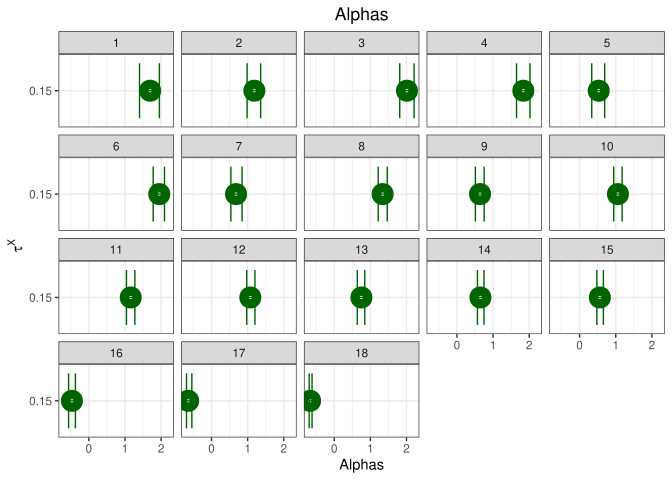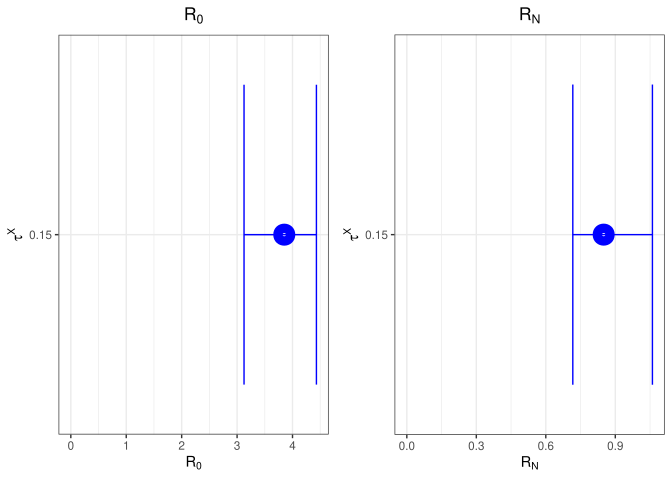## Sensitivity analysis

Here we perform the sensitivity analysis for $$\tau^{\mathcal{X}}$$ and $$\mu^{\mathcal{X}}$$.

### Natural spline

Results for various options for $$\tau^{\mathcal{X}}$$ (results in every row are for one value of the parameter):

0.15

First we show the estimated $$\alpha$$ values with 95% credible intervals:We also show the differences in $$R_t$$ for the different chosen parameters. We look at the mean $$R_t$$ with 95% credible interval in the time until first intervention ($$R_0$$) and after the last intervention ($$R_N$$):